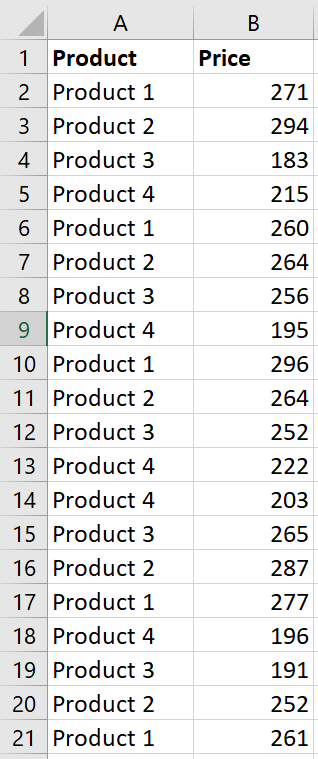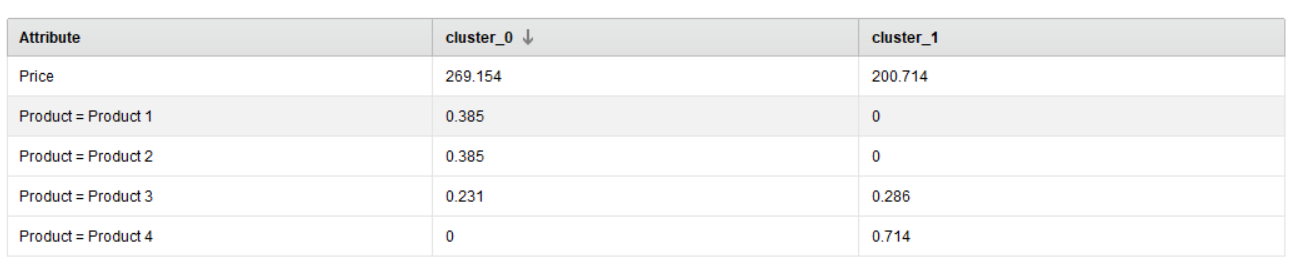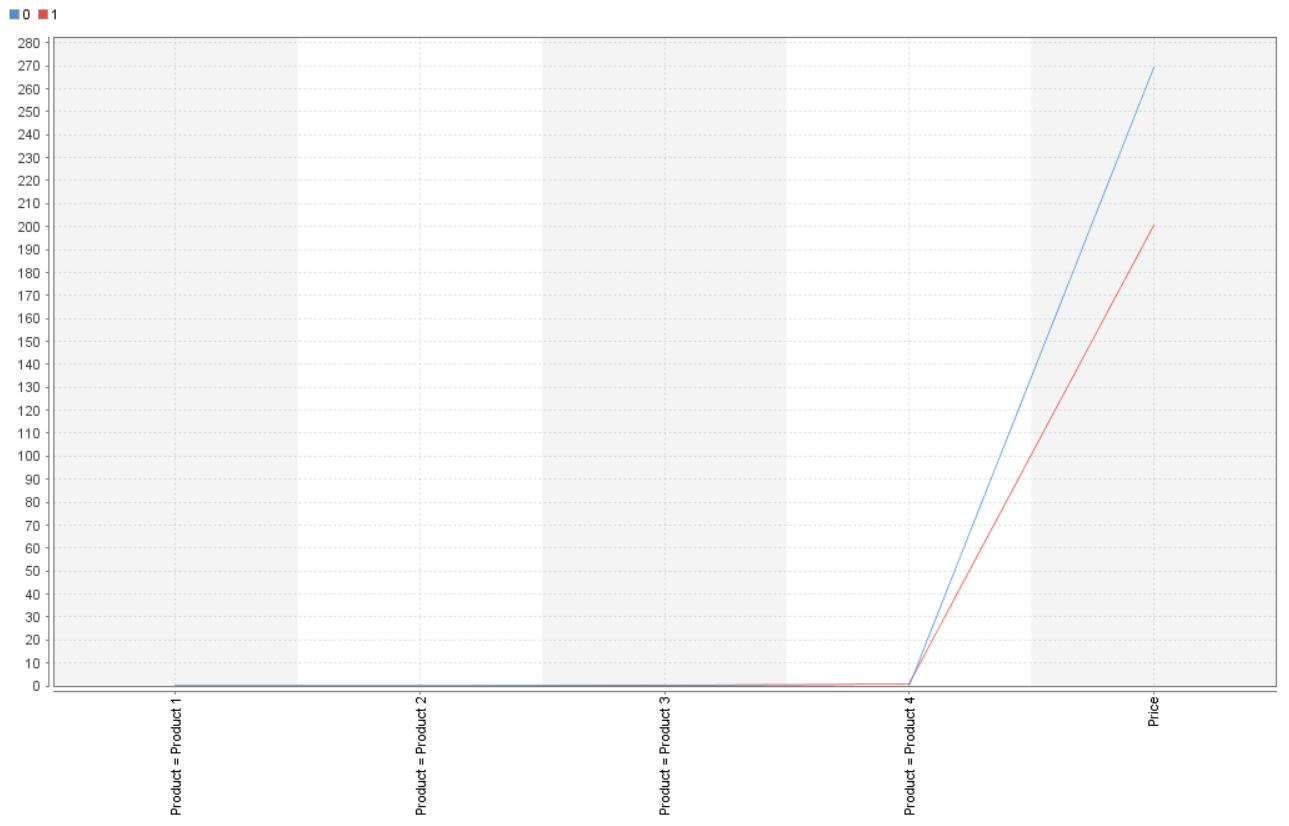# Detail Interpretation of K-means Centroid Table

Member Posts: 5Contributor I
edited June 2019 in Help

Hi everyone,

I know the concept of clustering and how it works. However, I am a little confused with the following case.

I have a dataset with only two features, product and price. The Product is categorical with limited possible values. The Price  is continous and represents products prices.Basic statistical analysis classify Product 1 and Product 2 into a cluster of high prices; and Product 3 and Product 4 into another cluster of low prices. Out of curiousity I just wanted to understand this case using clustering techniques in Rapidminer. Therefore, I created the following process.

`<?xml version="1.0" encoding="UTF-8"?><process version="8.2.000">  <context>    <input/>    <output/>    <macros/>  </context>  <operator activated="true" class="process" compatibility="8.2.000" expanded="true" name="Process">    <process expanded="true">      <operator activated="true" class="retrieve" compatibility="8.2.000" expanded="true" height="68" name="Retrieve products" width="90" x="45" y="85">        <parameter key="repository_entry" value="../data/products"/>      </operator>      <operator activated="true" class="nominal_to_numerical" compatibility="7.1.001" expanded="true" height="103" name="Nominal to Numerical" width="90" x="246" y="85">        <list key="comparison_groups"/>      </operator>      <operator activated="true" class="concurrency:k_means" compatibility="8.2.000" expanded="true" height="82" name="Clustering" width="90" x="447" y="85">        <parameter key="max_runs" value="50"/>      </operator>      <operator activated="true" class="extract_prototypes" compatibility="8.2.000" expanded="true" height="82" name="Extract Cluster Prototypes" width="90" x="581" y="85"/>      <connect from_op="Retrieve products" from_port="output" to_op="Nominal to Numerical" to_port="example set input"/>      <connect from_op="Nominal to Numerical" from_port="example set output" to_op="Clustering" to_port="example set"/>      <connect from_op="Clustering" from_port="cluster model" to_op="Extract Cluster Prototypes" to_port="model"/>      <connect from_op="Extract Cluster Prototypes" from_port="example set" to_port="result 1"/>      <connect from_op="Extract Cluster Prototypes" from_port="model" to_port="result 2"/>      <portSpacing port="source_input 1" spacing="0"/>      <portSpacing port="sink_result 1" spacing="0"/>      <portSpacing port="sink_result 2" spacing="0"/>      <portSpacing port="sink_result 3" spacing="0"/>    </process>  </operator></process>`

The above process produces the Centroid Table below.The followings are my questions, please bear with me if my questions are basic:

1. What are the values of cluster_0 and cluster_1 columns and how to interpret them?
2. Can we compare and interpret if cluster_0's value > cluster_1's value for a particular product, then that product belongs to cluster_0, otherwise it belongs to cluster_1?
3. My final question is how to intrepret the following plot that is produced by the process? and does it make sense to use clusering on such datasets with one or two categorical features and one continues feature?• Administrator, Moderator, Employee, RapidMiner Certified Analyst, RapidMiner Certified Expert, University Professor Posts: 3,453RM Data Scientist

Hi @wwahidi2018,

KMeans searches for the k means (or centers of gravity, if you want) in a data set. The table gives you the coordinates of these.

I think in your case you want to:

• Use k-Medoids and not k-means

K-Medoids ensures that the center is always an existing item. So you will get the most prototypical item as a center.

Cheers,

Martin

- Sr. Director Data Solutions, Altair RapidMiner -
Dortmund, Germany
• Member Posts: 5Contributor I

Hi @mschmitz,

Thanks a lot for your response.

I have problem understanding and interpreting values of the centroid table and if it is possible to compare those values as wrote my previous post? For example in the centrioid table attached to this post, is it possible to say Product 1 belongs to cluter_0 because the value for that product is 1?

I used normalized operator followed by k-Medoids operator as you advised. But still interpretation of the centroid table and plot confuse me in comparison to the basic statistics. Interpretation and justificaiton is easy using basic statistics for example based on the minimum price, maximum price, average price and so on.

`<?xml version="1.0" encoding="UTF-8"?><process version="8.2.000">  <context>    <input/>    <output/>    <macros/>  </context>  <operator activated="true" class="process" compatibility="8.2.000" expanded="true" name="Process">    <process expanded="true">      <operator activated="true" class="retrieve" compatibility="8.2.000" expanded="true" height="68" name="Retrieve products" width="90" x="45" y="85">        <parameter key="repository_entry" value="../data/products"/>      </operator>      <operator activated="true" class="normalize" compatibility="8.2.000" expanded="true" height="103" name="Normalize" width="90" x="179" y="187"/>      <operator activated="true" class="multiply" compatibility="8.2.000" expanded="true" height="103" name="Multiply" width="90" x="539" y="131"/>      <operator activated="true" class="nominal_to_numerical" compatibility="7.1.001" expanded="true" height="103" name="Nominal to Numerical" width="90" x="313" y="85">        <list key="comparison_groups"/>      </operator>      <operator activated="true" class="k_medoids" compatibility="8.2.000" expanded="true" height="82" name="Clustering" width="90" x="447" y="85"/>      <operator activated="true" class="extract_prototypes" compatibility="8.2.000" expanded="true" height="82" name="Extract Cluster Prototypes" width="90" x="581" y="85"/>      <connect from_op="Retrieve products" from_port="output" to_op="Normalize" to_port="example set input"/>      <connect from_op="Normalize" from_port="example set output" to_op="Multiply" to_port="input"/>      <connect from_op="Multiply" from_port="output 1" to_port="result 3"/>      <connect from_op="Multiply" from_port="output 2" to_op="Nominal to Numerical" to_port="example set input"/>      <connect from_op="Nominal to Numerical" from_port="example set output" to_op="Clustering" to_port="example set"/>      <connect from_op="Clustering" from_port="cluster model" to_op="Extract Cluster Prototypes" to_port="model"/>      <connect from_op="Extract Cluster Prototypes" from_port="example set" to_port="result 1"/>      <connect from_op="Extract Cluster Prototypes" from_port="model" to_port="result 2"/>      <portSpacing port="source_input 1" spacing="0"/>      <portSpacing port="sink_result 1" spacing="0"/>      <portSpacing port="sink_result 2" spacing="0"/>      <portSpacing port="sink_result 3" spacing="0"/>      <portSpacing port="sink_result 4" spacing="0"/>    </process>  </operator></process>`Why values for product 2 and 4 are zeros in both columns? Is it possible to say from this centroid if Product 2 and Product 4 is part of a cluster?What is the best way to explain this graph for the dataset?

• Administrator, Moderator, Employee, RapidMiner Certified Analyst, RapidMiner Certified Expert, University Professor Posts: 3,453RM Data Scientist

Hi,

you can read the table like this:

The most typical (=centroid) purchase (?) in cluster_0, is a purchase who bought a Product_1 with a normalized price of 0.5 (=high price). The most typical purchase in cluster_1 is a Product_3, with a low price.

Does this make more sense?

~Martin

- Sr. Director Data Solutions, Altair RapidMiner -
Dortmund, Germany
• Member Posts: 5Contributor I

Thank you now that makes more sense. However, it opens up another question such that what about the Product 2 and Product 4? In both column their values are 0.

Thank you,

• Administrator, Moderator, Employee, RapidMiner Certified Analyst, RapidMiner Certified Expert, University Professor Posts: 3,453RM Data Scientist

Hi,

that's the nature of k-medoids. It only gives yu a prototypical thing. I would in your case: Score your set and check which fraction of product_4's are in the clusters.

Best,

Martin

- Sr. Director Data Solutions, Altair RapidMiner -
Dortmund, Germany